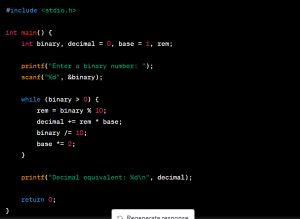Are you struggling with converting binary numbers to decimals in your C programming assignments? Don’t worry; we’ve got you covered! You can take c binary to decimal Assignment Help In this step-by-step guide, we will walk you through the process of converting binary numbers to decimals in a C program. So, let’s dive in and master this essential skill for your programming assignments.Before we start, it’s essential to understand the basics. The binary is a numbering system that uses only two digits: 0 and 1. On the other hand, decimal is a base-10 numbering system that uses ten digits from 0 to 9. Converting binary to decimal involves transforming binary numbers into their corresponding decimal equivalents.

Let’s begin by outlining the steps involved in converting binary to decimal in a C program:

## Step 1: Obtain the binary number

To start, you need to get the binary number from the user. You can use input functions like scanf() or command-line arguments to receive the binary number.

### Step 2: Initialize variables

Declare and initialize the variables required for the conversion process. You will need variables to store the binary number, the decimal equivalent, and the necessary counters.

### Step 3: Extract digits from the binary number

To convert binary to decimal, you need to extract the individual digits from the binary number. This can be done using a loop or by manipulating the digits using arithmetic operations.

#### Step 4: Calculate the decimal equivalent

Now that you have extracted the digits from the binary number, you can calculate the decimal equivalent. Multiply each binary digit by the corresponding power of 2 and sum them up to obtain the decimal value.

#### Step 5: Display the decimal value

Finally, display the calculated decimal value to the user. You can make use of output functions like printf() to showcase the result.

With these steps in mind, let’s take a look at a sample C program that converts binary to decimal:In the above program, we take the binary number as input from the user. Then, using a while loop, we extract the digits one by one and calculate the decimal value by multiplying each digit with the corresponding power of 2. Finally, we display the decimal equivalent.

Now that you have a clear understanding of the process, you can apply this knowledge to complete your binary-to-decimal conversion tasks in your programming assignments. However, if you still face any difficulties, there are various assignment help services available online that can provide assistance, such as “All Assignment Help” or “Do My Assignment.” These platforms offer expert guidance to help you overcome any challenges you may encounter.

In conclusion, converting binary to decimal in a C program may seem daunting at first, but by following the step-by-step guide provided above, you can confidently tackle this task. Remember to obtain the binary number, initialize variables, extract digits, calculate the decimal value, and display the result. With practice and persistence, you’ll become proficient in binary to decimal conversions, making your programming assignments a breeze.